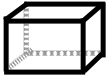## Surface Area of a Rectangular Prism

### Surface Area: Learn

Surface Area is the combined area of all two-dimensional surfaces of a shape. Just like ordinary area, the units are the squares of the units of length (that is, if a shape's sides are measured in meters, then the shape's area is measured in square meters).

A rectangular prism has six faces (flat surfaces), but they are not all identical. The opposite faces are identical, which means we have 3 sets of 2 faces to calculate, and then we add them all together.

For example, here is a rectangular prism with side lengths of 3m, 4m, and 5m.Each face of the prism has an area that is some combination of 3*4, 4*5, or 3*5. To account for all six faces, we need two copies of each combination, all added together:

 Total: 3*4 *2 = 24m2 4*5 *2 = 40m2 3*5 *2 = 30m2 94m2

### Surface Area: Practice

00:00

What is the surface area of a prism whose side lengths are
cm, cm, and cm?

Press the Start Button To Begin

You have 0 correct and 0 incorrect.

This is 0 percent correct.

### Play

Game Name Description Best Score
How many correct answers can you get in 60 seconds? 0
Extra time is awarded for each correct answer.
Play longer by getting more correct.
0
How fast can you get 20 more correct answers than wrong answers? 999